06-18-23, 09:48 PM   #1
Galseb
A Defias Bandit
Join Date: Jun 2023
Posts: 2Addon does not display messages in chat

In general, in a nutshell. I wanted to make an addon on WotLK, which does the calculation of the number of circles in Dalaran. But the problem is that when you enter the command, it writes that it is unknown
code:
Code:
```lua
local points = {
A = {x1 = 58, y1 = 46, x2 = 80, y2 = 49},
B = {x1 = 47, y1 = 28, x2 = 49, y2 = 36},
C = {x1 = 39, y1 = 48, x2 = 47, y2 = 52},
D = {x1 = 50, y1 = 58, x2 = 52, y2 = 63},
}
local currentPoint = "A"
local lapCount = 0
local DalaranID = 4395

SLASH_LAPCOUNT1 = "/lapcount"

lua
function SlashCmdList.LAPCOUNT(msg, editbox
print(lapCount)
end
end

local function CheckPlayerPosition()
local x, y = GetPlayerMapPosition("player")
local mapId = GetCurrentMapAreaID()

if mapId ~= DalaranID then
return
end

if x >= points[currentPoint].x1 and x <= points[currentPoint].x2 and y >= points[currentPoint].y1 and y <= points[currentPoint].y2 then
if currentPoint == "A" then
currentPoint = "B"
elseif currentPoint == "B" then
currentPoint = "C"
elseif currentPoint == "C" then
currentPoint = "D"
elseif currentPoint == "D" then
currentPoint = "A"
lapCount = lapCount + 1
end
end
end

local frame = CreateFrame("Frame")
frame:SetScript("OnUpdate", CheckPlayerPosition)```
I have a problem with the output of information, the game does not register the command. Help me fix the code please. ty for attention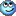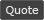06-19-23, 02:22 AM   #2
Dridzt
A Pyroguard Emberseer
Join Date: Nov 2005
Posts: 1,354
 Originally Posted by Galseb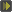In general, in a nutshell. I wanted to make an addon on WotLK, which does the calculation of the number of circles in Dalaran. But the problem is that when you enter the command, it writes that it is unknown code: Code: ```local points = { A = {x1 = 58, y1 = 46, x2 = 80, y2 = 49}, B = {x1 = 47, y1 = 28, x2 = 49, y2 = 36}, C = {x1 = 39, y1 = 48, x2 = 47, y2 = 52}, D = {x1 = 50, y1 = 58, x2 = 52, y2 = 63}, } local currentPoint = "A" local lapCount = 0 local DalaranID = 125 SLASH_LAPCOUNT1 = "/lapcount" function SlashCmdList.LAPCOUNT(msg, editbox) print(lapCount) end local function CheckPlayerPosition() local mapId = C_Map.GetBestMapForUnit("player") if mapId ~= DalaranID then return end local x, y = C_Map.GetPlayerMapPosition(mapId, "player") if x >= points[currentPoint].x1 and x <= points[currentPoint].x2 and y >= points[currentPoint].y1 and y <= points[currentPoint].y2 then if currentPoint == "A" then currentPoint = "B" elseif currentPoint == "B" then currentPoint = "C" elseif currentPoint == "C" then currentPoint = "D" elseif currentPoint == "D" then currentPoint = "A" lapCount = lapCount + 1 end end end local frame = CreateFrame("Frame") frame:SetScript("OnUpdate", CheckPlayerPosition)``` I have a problem with the output of information, the game does not register the command. Help me fix the code please. ty for attentionI fixed the problems visible to the naked eye (no testing)
You need to enable Lua errors from interface options to even see what's happening.
There's no way that code even run.06-19-23, 04:08 AM   #3
Galseb
A Defias Bandit
Join Date: Jun 2023
Posts: 2
 Originally Posted by DridztI fixed the problems visible to the naked eye (no testing) You need to enable Lua errors from interface options to even see what's happening. There's no way that code even run.
The output is now working, at least 0 shows, thanks!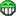But it does not count, and shows such script errors, the number of which is growing every second .. Can you tell me how to fix it? Thank youCode:
```Message: ...ace\AddOns\DalaranLoopCounter\DalaranLoopCounter.lua:18: attempt to call field 'GetBestMapForUnit' (a nil value)
Time: 06/19/23 13:00:02
Count: 139

Locals: (*temporary) = nil
(*temporary) = "player"
(*temporary) = "attempt to call field 'GetBestMapForUnit' (a nil value)"
DalaranID = 125
points = <table> {
A = <table> {
}
D = <table> {
}
C = <table> {
}
B = <table> {
}
}
currentPoint = "A"
lapCount = 0```
Lines 17 and 18 are
Code:
```local function CheckPlayerPosition()
local mapId = C_Map.GetBestMapForUnit("player")```06-19-23, 04:52 AM #4 Dridzt A Pyroguard Emberseer Join Date: Nov 2005 Posts: 1,354 Ah.. well the only possible reason for that error is you're not playing on official servers. Which means you can't get help on this forum.WoWInterface » Addon does not display messages in chat

 Thread Tools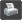Show Printable Version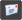Email this Page Display Modes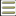Linear Mode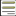Switch to Hybrid Mode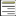Switch to Threaded Mode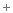Posting Rules You may not post new threads You may not post replies You may not post attachments You may not edit your posts vB code is On Smilies are On [IMG] code is On HTML code is Off